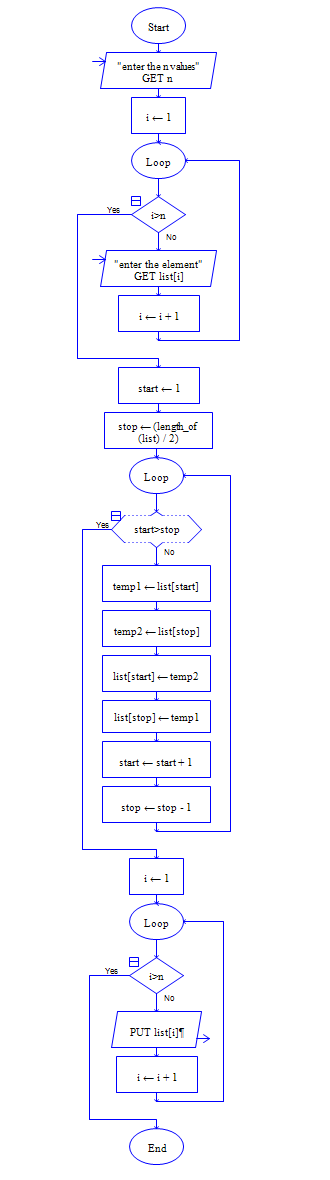# C Program to Reverse the Elements in array

Get array size n and n elements of array, then reverse the n elements.

Sample Input 1:

5 5 7 9 3 1

Sample Output 1:

1 3 9 7 5

#### Flow Chart Design#### Program or Solution

``` #include <stdio.h> #include <stdlib.h> int main(void) { int *a,n,i,j,temp; printf("Enter size of array:"); scanf("%d",&n); a=malloc(sizeof(int)*n); printf("Enter %d Elements:",n); for(i=0;i<n;i++) { scanf("%d",&a[i]); } for(i=0,j=n-1;i<j;i++,j--) { temp=a[i]; a[i]=a[j]; a[j]=temp; } printf("\After reversing the array:\n"); for(i=0;i<n;i++) { printf("%d ",a[i]); } return 0; } ```

#### Program Explanation

initialize i to first location of array and j to last location of array using i=0 j=n-1

swap the elements in location i and j, then increment i by 1 and decrement j by 1.

t=a[i] a[i]=a[j] a[j]=temp repeat the above step till i is less than j